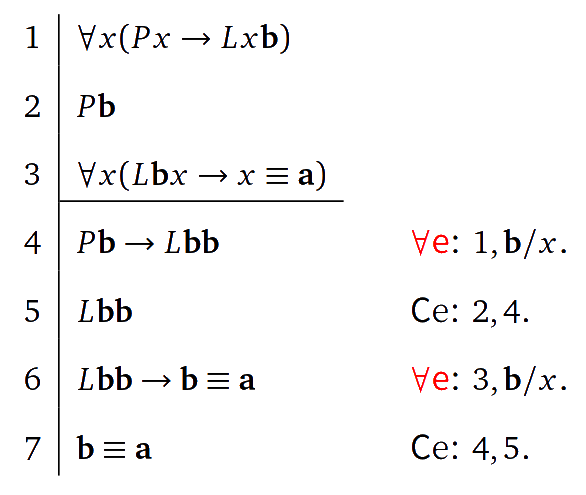# 1 全书绪论“I know what you’re thinking about,” said Tweedledum; “but it isn’t so, nohow.” “Contrariwise,” continued Tweedledee, “if it was so, it might be; and if it were so, it would be; but as it isn’t, it ain’t. That’s logic.”Lewis Carroll, Through the Looking-Glass, 1872

1. 什么是 $$X$$？（$$X$$ 的定义）
2. 学好 $$X$$ 在理论上有什么意义？
3. 学好 $$X$$ 在实践上有什么意义？
4. 怎样学好 $$X$$？……

1. 逻辑学是关于论说（argument）的。
2. 逻辑学是关于推理（inference, reasoning）的。
3. 逻辑学是关于理论或信念的一致性（consistency）的。

## 1.1 论说

1. 人人都爱我的宝宝。
2. 我的宝宝只爱我。

1. 人人都爱我的宝宝。
2. 我的宝宝只爱我。
3. 所以，我的宝宝就是我。

### 1.1.2 论说形式的好坏

1. 企鹅都是鸟。
2. 猴子都不是企鹅。
3. 所以，猴子都不是鸟。

1. 爱运动的学生学习成绩都很好。
2. 男学生都爱运动。
3. 所以，男学生学习成绩都很好。

1. 所有的猫都是人。
2. 所有的学生都是猫。
3. 所以，所有的学生都是人。

1. 所有的猫都是人。
2. 张三是猫。
3. 所以，张三是人。

1. 所有 $$S$$$$P$$
2. $$a$$$$S$$
3. 所以，$$a$$$$P$$

1. 所有 $$S$$$$P$$
2. 所有 $$Q$$ 都不是 $$S$$
3. 所以，所有 $$Q$$ 都不是 $$P$$

1. 所有 $$S$$$$P$$
2. 所有 $$Q$$$$S$$
3. 所以，所有 $$Q$$$$P$$

1. 企鹅都是鸟。
2. 猴子都不是企鹅。
3. 所以，猴子都不是鸟。

1. 企鹅都是鸟。
2. 麻雀都不是企鹅。
3. 所以，麻雀都不是鸟。

### 1.1.3 论说好坏取决于形式的好坏

1. 浩毕特都生活在沙伊鄂。
2. 拜根斯都是浩毕特。
3. 所以，拜根斯都生活在沙伊鄂。

1. 所有 $$S$$$$P$$
2. 所有 $$Q$$$$S$$
3. 所以，所有 $$Q$$$$P$$

## 1.2 演绎

### 1.2.1 演绎的例子一

1. 所有的人都爱我的宝宝。 前提（经过复述）
2. 我的宝宝是人。 预设
3. 我的宝宝爱我的宝宝。 三段论
4. 我的宝宝只爱我。 前提
5. 对每个 $$x$$，如果我的宝宝爱 $$x$$，那么 $$x$$ 就是我。 「按定义改写」
6. 如果我的宝宝爱我的宝宝，那么我的宝宝就是我。 特例
7. 我的宝宝就是我。 肯定前件的假言推理（modus ponens）「所有的人」的这种「…以外的所有的人」的用法，是个习惯或语境的问题，而非命题的逻辑结构或推理的问题。显然，我们不能说「所有的人」的意思就是「宝宝以外的所有的人」的意思，因为在「所有的人都要睡觉」「所有的人都要吃饭」等句子中，「所有的人」显然不是指宝宝以外的所有的人。当然我们就更不能说「所有的 $$S$$$$P$$」中的「所有的 $$S$$」应理解为宝宝以外的所有的 $$S$$。如果把「所有的人都爱我的宝宝」的形式概括为「所有的 $$S$$ 都与 $$a$$ 有关系 $$R$$」（其中 $$a$$ 是某个 $$S$$，就像我的宝宝是人），那么「所有的 $$S$$」在句子中的意思是否就是所有不等于 $$a$$$$S$$ 呢？有些时候是这样，但显然也并不总是这样。

1. 所有的 $$S$$ 都与 $$a$$ 有关系 $$R$$，除了 $$a$$.
2. $$a$$$$S$$ 且只与 $$b$$ 有关系 $$R$$.

### 1.2.2 演绎的例子二

1. 2+2=5 假设
2. 2=3 等词法则（等式两边各减 2）
3. 3=2 等词法则（对称性）
4. 2=1 等词法则（等式两边各减 1）
5. 罗素和教皇是两个人。 事实
6. 罗素和教皇是同一个人。 等词法则（运用上述「2=1」）

## 1.3 一致性

### 1.3.1 关于一致性的基本想法

1. 相对于给定的演绎系统，如果语义一致的理论都是语法一致的，那么该系统一定有「可靠性」，亦即从前提可演绎的都是这些前提的逻辑后承。
2. 相对于给定的演绎系统，如果语法一致的理论都是语义一致的，那么该系统一定有「完全性」，亦即只要是前提的逻辑后承都从这些前提可演绎。（：这一节中有几个命题，其真实性对初学者来说可能不那么明显。我们并不打算在此处详细讨论这些命题，而会在后面讨论它们。所以，即使现在不明白这些命题为什么成立，初学者也不必因此而对逻辑心生畏惧；读完这本讲义后回头来看这些命题时，就会明白它们成立的理由了。）

### 1.3.2 不一致命题集的例子

1. 海德格尔是纳粹。
2. 海德格尔不是纳粹。

1. 吾盾之坚，物莫能陷也。
2. 吾矛之利，于物无不陷也。

1. 这位理发师只为那些不为自己理发的人理发（即不为任何为自己理发的人理发）。
2. 这位理发师为所有不为自己理发的人理发。

1. 哲学家都是求真的人。
2. 求真的人都不喜欢神侃。
3. 张三是哲学家。
4. 张三喜欢神侃。

### 1.3.3 一致性、逻辑蕴涵与可演绎性

1. 如果一个理论不一致，那么对其中的任何命题，该理论的其他命题一定逻辑蕴涵它的否定。
2. 如果一个理论是一致的，那么对其中的任何命题，该理论的其他命题不会逻辑蕴涵它的否定。
3. 如果一个理论不一致，那么对其中的任何命题，它的否定从该理论的其他命题一定可演绎。
4. 如果一个理论是一致的，那么对其中的任何命题，它的否定从该理论的其他命题一定不可演绎。（：把一致性理解为语义一致性时，上述第三个命题等价于「完全性定理」，第四个等价于「可靠性定理」；而把一致性理解为语法一致性时，上述第一个命题等价于「可靠性定理」，第二个等价于「完全性定理」（后两个合起来是定理334）。）

## 1.4 逻辑或「逻辑」的若干问题

### 1.4.1 「逻辑是什么」不是逻辑问题

「逻辑是什么」这类问题，当然可以讨论。这样的讨论有两种。一是随便谈谈看法，二是认真的学术讨论。如果是随便谈看法，即使是外行来谈，只要谈的不是太离谱人们也不会去较真，因为任何人都可以有自己的看法。（：“Few men think, yet all will have opinions?” — George Berkeley, Three Dialogues between Hylas and philonous, 1713.）认真的学术讨论则不同，它决不像外行或初学者想像的那么简单，而且即使讨论者的态度是十二分的认真，也未必能使人严肃认真地对待他们的讨论。这是因为，要认真讨论「逻辑是什么」这类问题，首先要熟悉逻辑，要有较全面的学识和较好的修养。倘若只读了几本逻辑教科书，甚至连像样的教课书都没好好读过，那么讨论「逻辑是什么」这类问题是不会得要领的，从而无法指望内行人认真对待这样的讨论。

### 1.4.2 逻辑与「逻辑」的用法

「逻辑」的诸多用法显然无法对应于「专家」说的那五六种逻辑，这一事实不仅无法否认，而且与「专家」的前提和结论无法同世而立。所以，如果我们的「专家」不肯放弃他的前提或结论，那就只会让自己落到全无逻辑的地步。（这也是「逻辑」的一种用法!）

### 1.4.4 当代逻辑、传统逻辑和「普通人需要的逻辑」

「批判性思维」之类的东西，作为一种初级逻辑的教学模式，或者作为关于初级逻辑的普及和日常应用的探索，是值得鼓励的。在美国不少大学，「逻辑导论」课是通选课，虽俗称「baby logic」，但仍有不少学生难以过关；而「批判性思维」课（如果有的话），俗称「baby baby logic」，就是专为这些学生开设的。国内不少学生甚至学者在逻辑性方面的欠缺是严重的，而普通的「逻辑导论」课又似乎对他们帮助不大；因而就逻辑的教学普及而言，我赞成借鉴甚至进口「批判性思维」这类课程。（二狗：批判性思维：foetus logic；逻辑导论：baby logic；经典数理逻辑：basic logic.）但是，就逻辑理论而言，「新逻辑」并不是新逻辑，因为无论人们的愿望是什么，它尚未提供能与当代逻辑理论相比的逻辑理论。